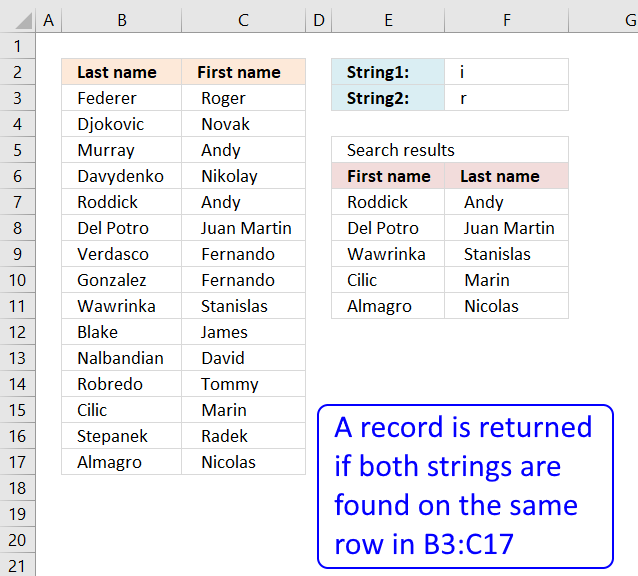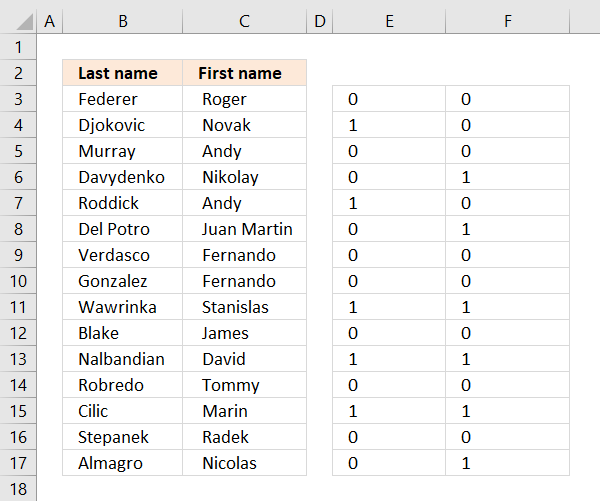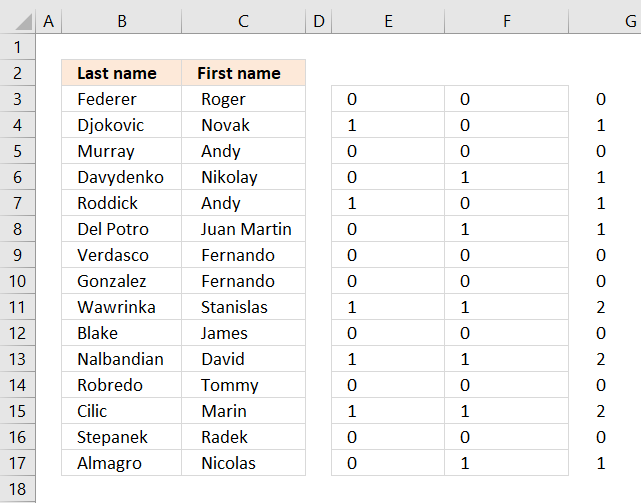Author: Oscar Cronquist Article last updated on August 11, 2019### Question:

I want to search two columns with two search strings? The strings can be anywhere in these two columns but they both have to be somewhere on the same row to match. The search is not case sensitive.

### Array formula in cell D7:

=INDEX(\$B\$3:\$C\$17, SMALL(IF((MMULT(ISNUMBER(SEARCH(\$F\$2, \$B\$3:\$C\$17))*1, {1;1})>0)*(MMULT(ISNUMBER(SEARCH(\$F\$3, \$B\$3:\$C\$17))*1, {1;1})>0), MATCH(ROW(\$B\$3:\$C\$17), ROW(\$B\$3:\$C\$17)), ""), ROWS(\$A\$1:A1)), COLUMNS(\$A\$1:A1))

How to create an array formula

1. Select cell D7
2. Press with left mouse button on in formula bar
3. Copy and paste array formula to formula bar
4. Press and hold Ctrl + Shift
5. Press Enter
6. Release all keys

How to copy an array formula

1. Select cell D7
2. Copy the cell (Ctrl + c)
3. Select cell range D7:D12
4. Paste (Ctrl + v)
5. Copy cell range D7:D12 (Ctrl + c)
6. Select cell range E7:E12
7. Paste (Ctrl + v)

### Explaining formula in cell D7

#### Step 1 - Search \$B\$3:\$C\$17 for value in cell \$F\$2

The SEARCH function returns the relative position of the search string, if nothing found then the function returns an #VALUE! error.

SEARCH(\$F\$2,\$B\$3:\$C\$17)

becomes

SEARCH("'i",{"Federer"," Roger "; ... ," Nicolas "})

and returns

{#VALUE!,#VALUE!; 7,#VALUE!; #VALUE!,#VALUE!; #VALUE!,3; 5,#VALUE!; #VALUE!,11; #VALUE!,#VALUE!; #VALUE!,#VALUE!; 5,6; #VALUE!,#VALUE!; 8,5; #VALUE!,#VALUE!; 2,5; #VALUE!,#VALUE!; #VALUE!,3}

#### Step 2 - Convert array to boolean values

The ISNUMBER function coonverts errors into TRUE and remaining values into FALSE.

ISNUMBER(SEARCH(\$F\$2,\$B\$3:\$C\$17))*1

becomes

ISNUMBER({#VALUE!,#VALUE!; 7,#VALUE!; #VALUE!,#VALUE!; #VALUE!,3; 5,#VALUE!; #VALUE!,11; #VALUE!,#VALUE!; #VALUE!,#VALUE!; 5,6; #VALUE!,#VALUE!; 8,5; #VALUE!,#VALUE!; 2,5; #VALUE!,#VALUE!; #VALUE!,3})*1

becomes

{FALSE,FALSE;TRUE, FALSE; FALSE,FALSE;FALSE, TRUE;TRUE,FALSE; FALSE,TRUE; FALSE,FALSE; FALSE,FALSE; TRUE,TRUE; FALSE,FALSE; TRUE,TRUE; FALSE,FALSE; TRUE,TRUE; FALSE,FALSE; FALSE,TRUE}*1

The MMULT function can't work with boolean values, we must multiply with 1 to convert boolean values into numerical equivalents:

{0,0;1,0;0,0;0, 1;1,0;0,1; 0,0;0,0;1, 1;0,0;1,1; 0,0;1,1;0, 0;0,1}#### Step 3 - Sum values on each row

The MMULT function sums values row-wise.

MMULT(ISNUMBER(SEARCH(\$F\$2,\$B\$3:\$C\$17))*1,{1;1})>0

becomes

MMULT({0,0;1,0;0,0;0, 1;1,0;0,1; 0,0;0,0;1, 1;0,0;1,1; 0,0;1,1;0, 0;0,1},{1;1})

becomes

{0;1;0;1;1;1;0;0;2;0;2;0;2;0;1}>0

and returns

{FALSE;TRUE; FALSE;TRUE; TRUE;TRUE; FALSE;FALSE; TRUE;FALSE; TRUE;FALSE; TRUE;FALSE; TRUE}

The array is entered in column G, it is now very clear that MMULT function sums values on each row.#### Step 4 - Search string 2

This step demonstrates the same steps 1 to 3, however, the search string is in cell E3

(MMULT(ISNUMBER(SEARCH(\$E\$3,\$B\$3:\$C\$17))*1,{1;1})>0)

returns

{TRUE;FALSE; TRUE;FALSE; TRUE;TRUE; TRUE;TRUE; TRUE;FALSE; FALSE;TRUE; TRUE;TRUE; TRUE}

#### Step 5 - Multiply arrays

Both conditions must be met in other words both strings must have been found in a row, see table below.

 Boolean Boolean Result FALSE FALSE 0 TRUE FALSE 0 TRUE TRUE 1

(MMULT(ISNUMBER(SEARCH(\$L\$2,\$B\$3:\$C\$17))*1,{1;1})>0)*(MMULT(ISNUMBER(SEARCH(\$L\$3,\$B\$3:\$C\$17))*1,{1;1})>0)

becomes

{FALSE;TRUE; FALSE;TRUE; TRUE;TRUE; FALSE;FALSE; TRUE;FALSE; TRUE;FALSE; TRUE;FALSE; TRUE}*{TRUE;FALSE; TRUE;FALSE; TRUE;TRUE; TRUE;TRUE; TRUE;FALSE; FALSE;TRUE; TRUE;TRUE; TRUE}

and returns

{0;0; 0;0; 1;1; 0;0; 1;0; 0;0; 1;0; 1}

#### Step 6 - Replace TRUE with corresponding row number

IF((MMULT(ISNUMBER(SEARCH(\$L\$2,\$B\$3:\$C\$17))*1,{1;1})>0)*(MMULT(ISNUMBER(SEARCH(\$L\$3,\$B\$3:\$C\$17))*1,{1;1})>0),MATCH(ROW(\$B\$3:\$C\$17),ROW(\$B\$3:\$C\$17)),"")

becomes

IF({0;0; 0;0; 1;1; 0;0; 1;0; 0;0; 1;0; 1},MATCH(ROW(\$B\$3:\$C\$17),ROW(\$B\$3:\$C\$17)),"")

becomes

IF({0;0; 0;0; 1;1; 0;0; 1;0; 0;0; 1;0; 1},{1;2;3;4;5;6;7;8;9;10;11;12;13;14;15},"")

and returns

{"";"";"";"";5;6;"";"";9;"";"";"";13;"";15}.

#### Step 7 - Extract k-th smallest row number

SMALL(IF((MMULT(ISNUMBER(SEARCH(\$L\$2,\$B\$3:\$C\$17))*1,{1;1})>0)*(MMULT(ISNUMBER(SEARCH(\$L\$3,\$B\$3:\$C\$17))*1,{1;1})>0),MATCH(ROW(\$B\$3:\$C\$17),ROW(\$B\$3:\$C\$17)),""),ROWS(\$A\$1:A1))

becomes

SMALL({"";"";"";"";5;6;"";"";9;"";"";"";13;"";15},ROWS(\$A\$1:A1))

The ROWS function returns the number of rows in a cellreference, this cell reference expands when formula is copied to cells below. This makes sure a new row number is extracted and returned in each cell.

SMALL({"";"";"";"";5;6;"";"";9;"";"";"";13;"";15},1)

and returns 5.

#### Step 8 - Return value

The INDEX function gets a number based on row and column numbers.

INDEX(\$B\$3:\$C\$17, SMALL(IF((MMULT(ISNUMBER(SEARCH(\$F\$2, \$B\$3:\$C\$17))*1, {1;1})>0)*(MMULT(ISNUMBER(SEARCH(\$F\$3, \$B\$3:\$C\$17))*1, {1;1})>0), MATCH(ROW(\$B\$3:\$C\$17), ROW(\$B\$3:\$C\$17)), ""), ROWS(\$A\$1:A1)), COLUMNS(\$A\$1:A1))

becomes

INDEX(\$B\$3:\$C\$17, 5, COLUMNS(\$A\$1:A1))

The COLUMNS function keeps track of which value to return horizontally.

INDEX(\$B\$3:\$C\$17, 5, COLUMNS(\$A\$1:A1))

becomes

INDEX(\$B\$3:\$C\$17, 5, 1)

and returns "Roddick" in cell E7.

### Get Excel *.xlsx file

multiple-criteria-lookup-with-multiple-results-2v3.xlsx